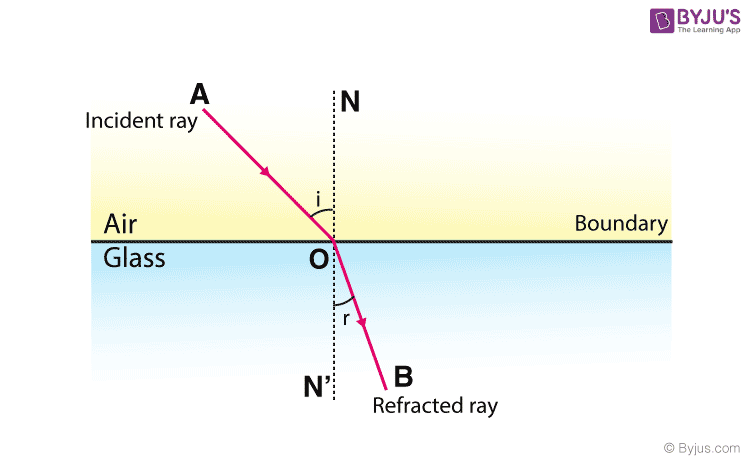Question

# State the laws of refraction.

Open in App
Solution

## Refraction: In physics, refraction is the change in direction of a wave travelling from one medium to another induced by a change in speed. According to Snell's laws or the law of refraction:The incident ray, the refracted ray, and the normal at the point of incidence, all lie in the same plane.The ratio of the sine of the angle of incidence ‘i’ to the sine of the angle of refraction ‘r’ is constant for the pair of given media is always constant.This constant is called the refractive index of the second medium w.r.t. the first medium and can be expressed as:${\mu }_{2}^{1}=\frac{\mathrm{sin}i}{\mathrm{sin}r}$Hence, the laws of refraction have been stated and explained.Suggest Corrections188Similar questions
Join BYJU'S Learning ProgramRelated VideosOptical Density
PHYSICS
Watch in AppExplore more
Join BYJU'S Learning Program